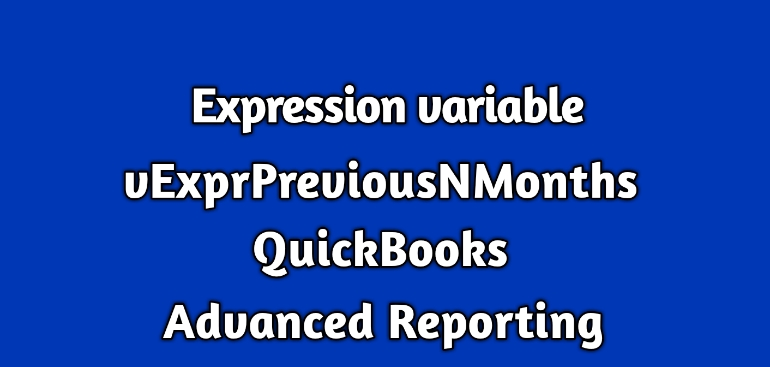# Expression variable (vExprPreviousNMonths) in QuickBooks Enterprise Advanced ReportingThe QuickBooks Advanced Reporting has many handy expression variables. Most of these variables are for dates.

This blog discusses vExprPreviousNMonths.

This expression variable is defined as

[Period ID] = {\$(=Max([Period ID]) – \$1)},Year=,Quarter=,[Quarter ID]=Month= ,Period= ,Week=,Day= ,[Transaction.Txn Date] =,[Month Year]=,[Quarter Year]=

Where to use it:

This expression variable is very helpful for a monthly sales report.

Here are expressions for the last two months of the current year and for the same months from the previous 2 years.

Expressions for the last two months of the current year:

sum({<\$(vExprSales),\$(vExprPreviousNMonths(0))>}[Transactions.Amount With Sign])

sum({<\$(vExprSales),\$(vExprPreviousNMonths(1))>}[Transactions.Amount With Sign])

Expressions for the last two months of the previous year:

sum({<\$(vExprSales),\$(vExprPreviousNMonths(11))>}[Transactions.Amount With Sign])

sum({<\$(vExprSales),\$(vExprPreviousNMonths(12))>}[Transactions.Amount With Sign])

Expressions for the last two months of the previous to previous year:

sum({<\$(vExprSales),\$(vExprPreviousNMonths(22))>}[Transactions.Amount With Sign])

sum({<\$(vExprSales),\$(vExprPreviousNMonths(23))>}[Transactions.Amount With Sign])

So, what is the variable in all these expressions?

It is these number 0, 1, 11, 12, etc. which is the parameter to the expression variable.

This number can be derived by subtracting 1 from the nth position of the month from the current month.

For example;

If the current month is July 2018 then the number that will be used as the parameter will for Nov 2016 be calculated as follows:

The November 2016 is at the 20th position from the current month. So, the number to use in the expression is 19.

sum({<\$(vExprSales),\$(vExprPreviousNMonths(19))>}[Transactions.Amount With Sign])

The best thing about this expression variable is that it takes care of change of the year.

Other usages:

You can use this expression to compare the sales between two months.

For instance, the below expression can be used to find the difference of sales amount between the current month and same month last year.

sum({<\$(vExprSales),\$(vExprPreviousNMonths(0))>}[Transactions.Amount With Sign]) –
sum({<\$(vExprSales),\$(vExprPreviousNMonths(11))>}[Transactions.Amount With Sign])

The expression for a label:

There is related expression variable to be used in the label.

It is vTxtMonthYear.

You can use the variable in the Label like

=\$(vTxtMonthYear(0))  for the current month.

=\$(vTxtMonthYear(1))  for the previous month and so on.